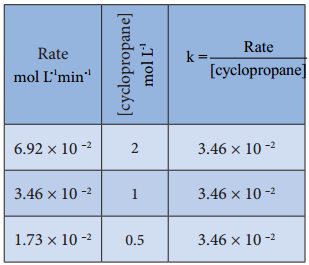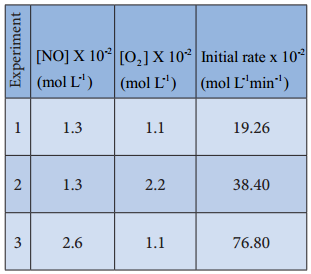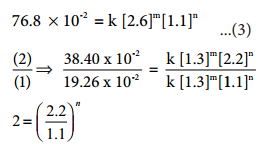Find free online Chemistry Topics covering a broad range of concepts from research institutes around the world.

## Rate Law and Rate Constant

Rate Law and Rate Constant:

We have just learnt that, the rate of the reaction depends upon the concentration of the reactant. Now let us understand how the reaction rate is related to concentration by considering the following general reaction.

xA + yB → products

The rate law for the above reaction is generally expressed as Rate = k [A]m[B]n

Where k is proportionality constant called the rate constant. The values of m and n represent the reaction order with respect to A and B respectively. The overall order of the reaction is given by (m+n). The values of the exponents (m and n) in the rate law must be determined by experiment. They cannot be deduced from the Stoichiometry of the reaction. For example, consider the isomerisation of cyclopropane, that we discussed earlier.

The results shown in table 7.2 indicate that if the concentration of cyclopropane is reduced to half, the rate also reduced to half. It means that the rate depends upon [cyclopropane] raised to the first power i.e., Rate = k[cyclopropane]1

⇒ $$\frac{Rate}{[cyclopropane]}$$ = k

Rate Constant for IsomerisationLet us consider an another example, the oxidation of nitric oxide (NO)

2NO(g) + O2(g) → 2NO2(g)

Series of experiments are conducted by keeping the concentration of one of the reactants constant and the changing the concentration of the others.Rate = k [NO]m[O2]n
For experiment 1, the rate law is
Rate1 = k [NO]m[O2]n
19.26 × 10-2 = k[1.3]m[1.1]n ………….. (1)
Similarly for experiment 2
Rate2 = k[NO]m[O2]n
38.40 × 10-2 = k[1.3]m[2.2]n …………… (2)
For experiment 3
Rate3 = k[NO]m[O2]n
76.8 × 10-2 = k[2.6]m[1.1]n …………….. (3)2=2n i.e., n = 1

Therefore the reaction is first order with respect to O24=2m i.e., m = 2

Therefore the reaction is second order with respect to NO

The rate law is Rate1 = k[NO]2[O2]1
The overall order of the reaction = (2 + 1) = 3

Differences Between Rate and Rate Constant of a Reaction:

 Rate of a Reaction Rate Constant of a Reaction 1. It represents the speed at which the reactants are converted into products at any instant. 1. It is a proportionality constant. 2. It is measured as decrease in the concentration of the reactants or increase in the concentration of products. 2. It is equal to therate of reaction, when the concentration of each of the reactants is unity 3. It depends on the initial concentration of reactants. 3. It does not depend on the initial concentration of reactants.